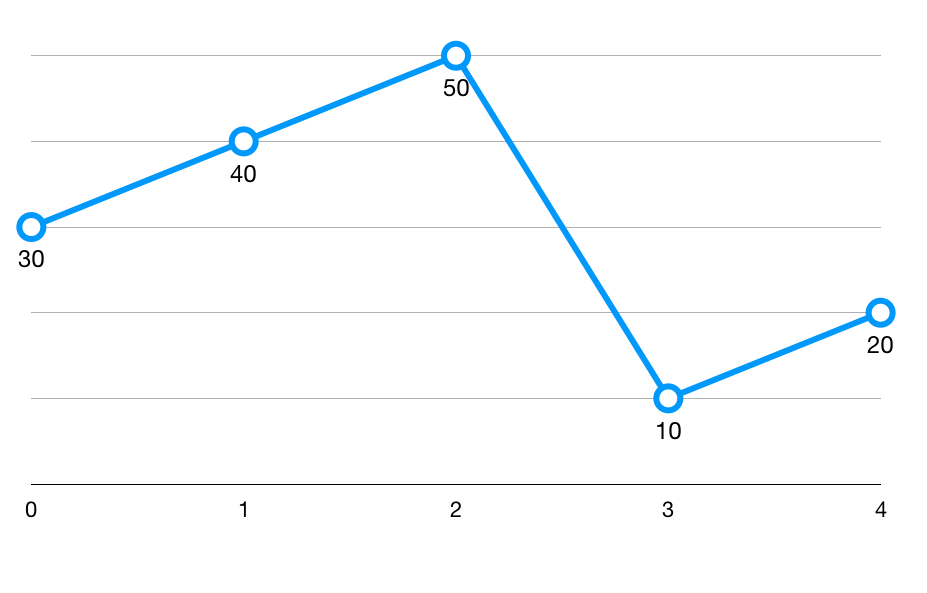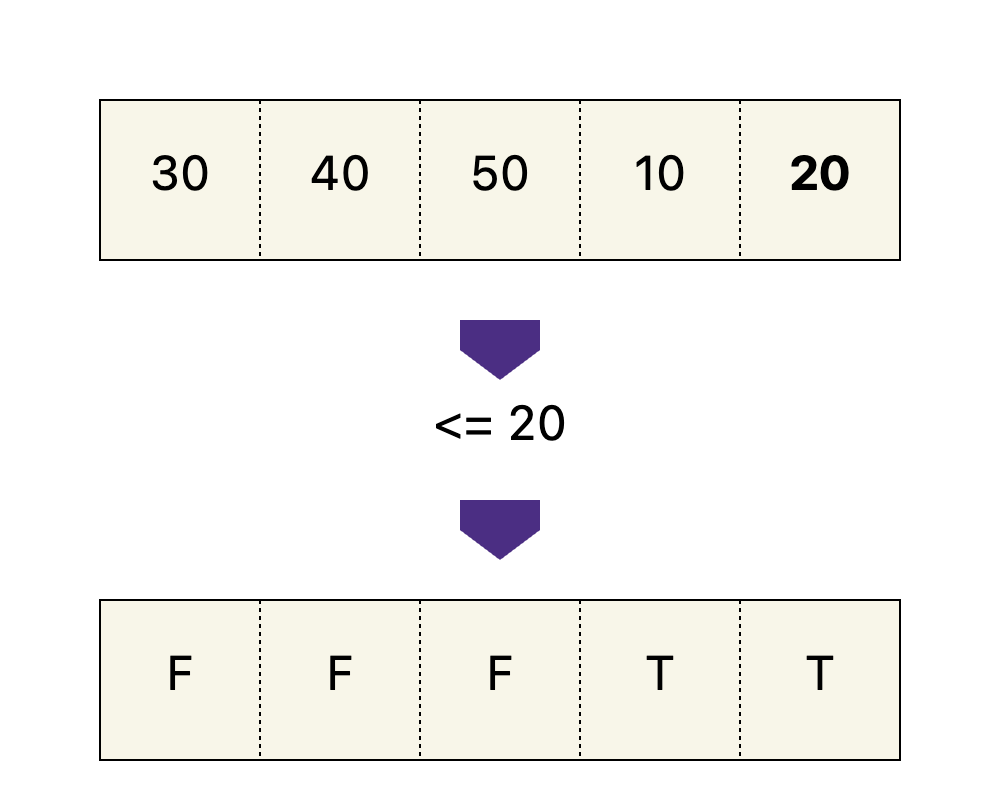# Find Minimum in Rotated Sorted Array

A sorted array of unique integers was rotated at an unknown pivot. For example, `[10, 20, 30, 40, 50]` becomes `[30, 40, 50, 10, 20]`. Find the index of the minimum element in this array. All the numbers are unique.

Input: `[30, 40, 50, 10, 20]`

Output: `3`

Explanation: the smallest element is 10 and its index is 3.

Input: `[3, 5, 7, 11, 13, 17, 19, 2]`

Output: `7`

Explanation: the smallest element is 2 and its index is 7.

## Explanation

At first glance, it seems that there's no way to do it in less than linear time. The array is not sorted.

But remember binary search can work beyond sorted arrays, as long as there is a binary decision we can use to shrink the search range.

Let's draw a figure and see if there's any pattern. If we plot the numbers against their index, we get:Notice the numbers are divided into two sections: numbers larger than the last element of the array and numbers smaller than it. The minimum element is at the boundary between the two sections.

We can apply a filter of `< the last element` and get the boolean array that characterizes the two sections.Now the problem is yet again reduced to finding the first `true` element in a boolean array. And we already know how to do this from Find the Boundary module.

Time Complexity: `O(log(n))`

``````1from typing import List
2
3def find_min_rotated(arr: List[int]) -> int:
4    left, right = 0, len(arr) - 1
5    boundary_index = -1
6
7    while left <= right:
8        mid = (left + right) // 2
9        # if <= last element, then belongs to lower half
10        if arr[mid] <= arr[-1]:
11            boundary_index = mid
12            right = mid - 1
13        else:
14            left = mid + 1
15
16    return boundary_index
17
18if __name__ == '__main__':
19    arr = [int(x) for x in input().split()]
20    res = find_min_rotated(arr)
21    print(res)
22``````